Examples

Chapter 14 Class 10 Statistics
Serial order wise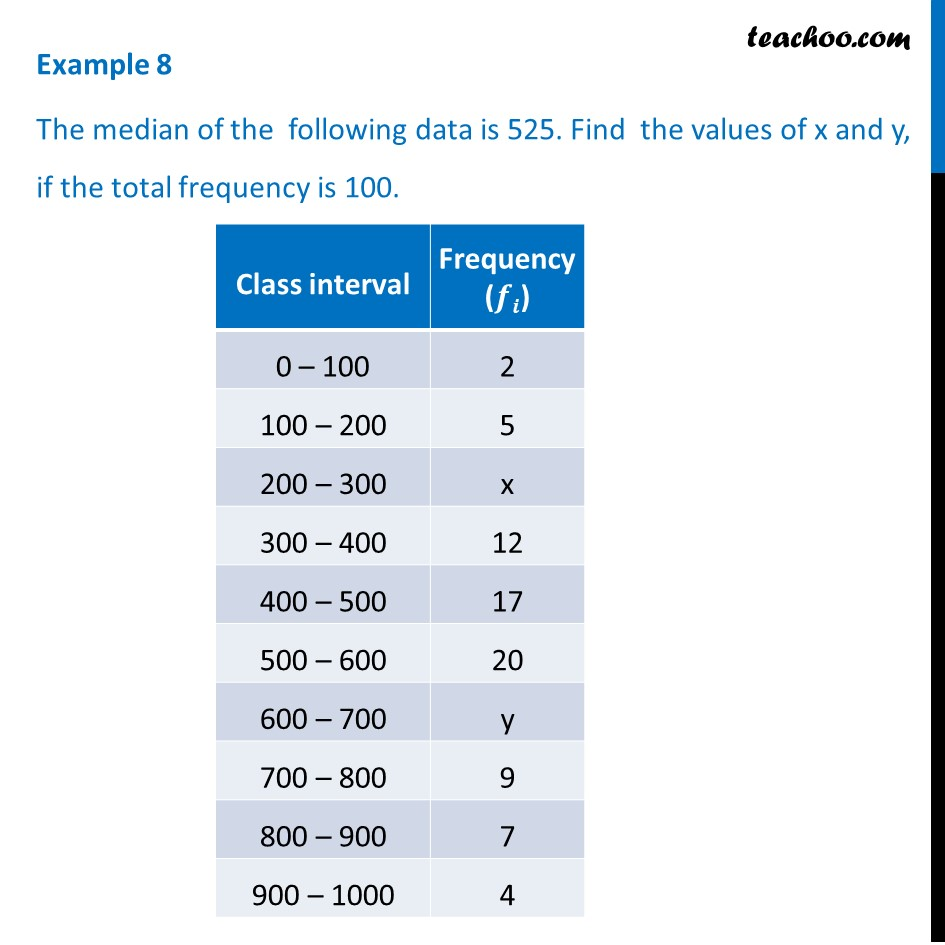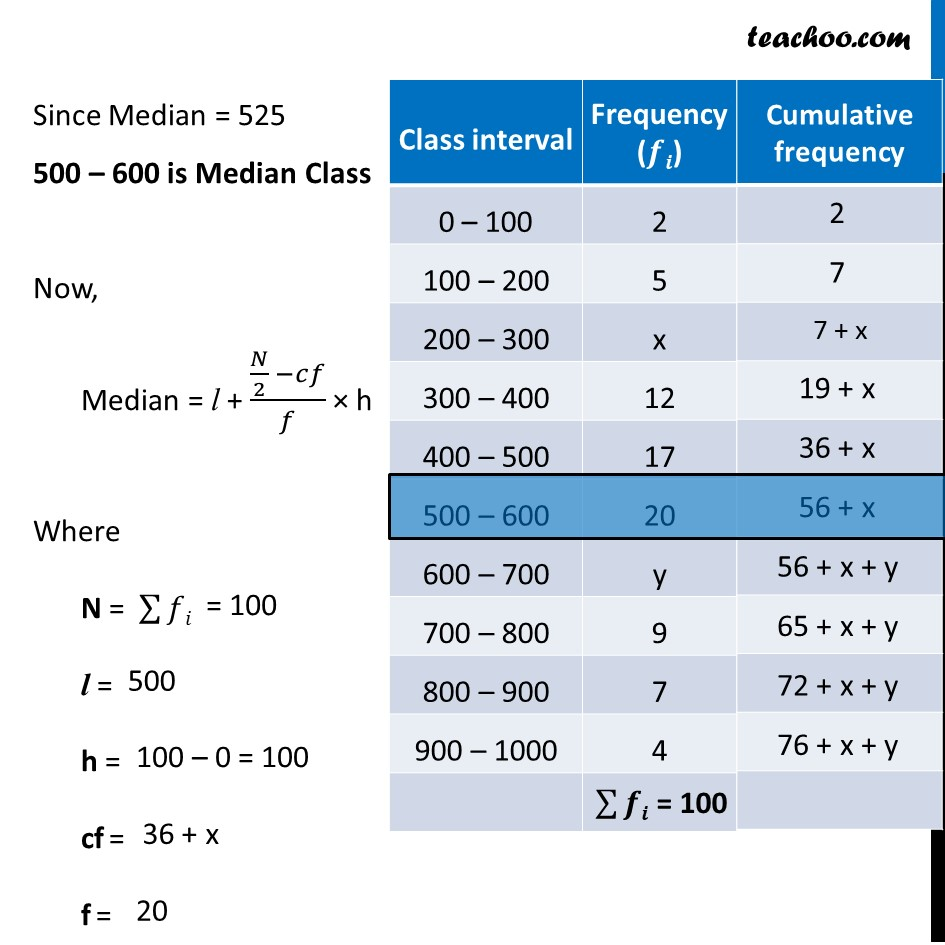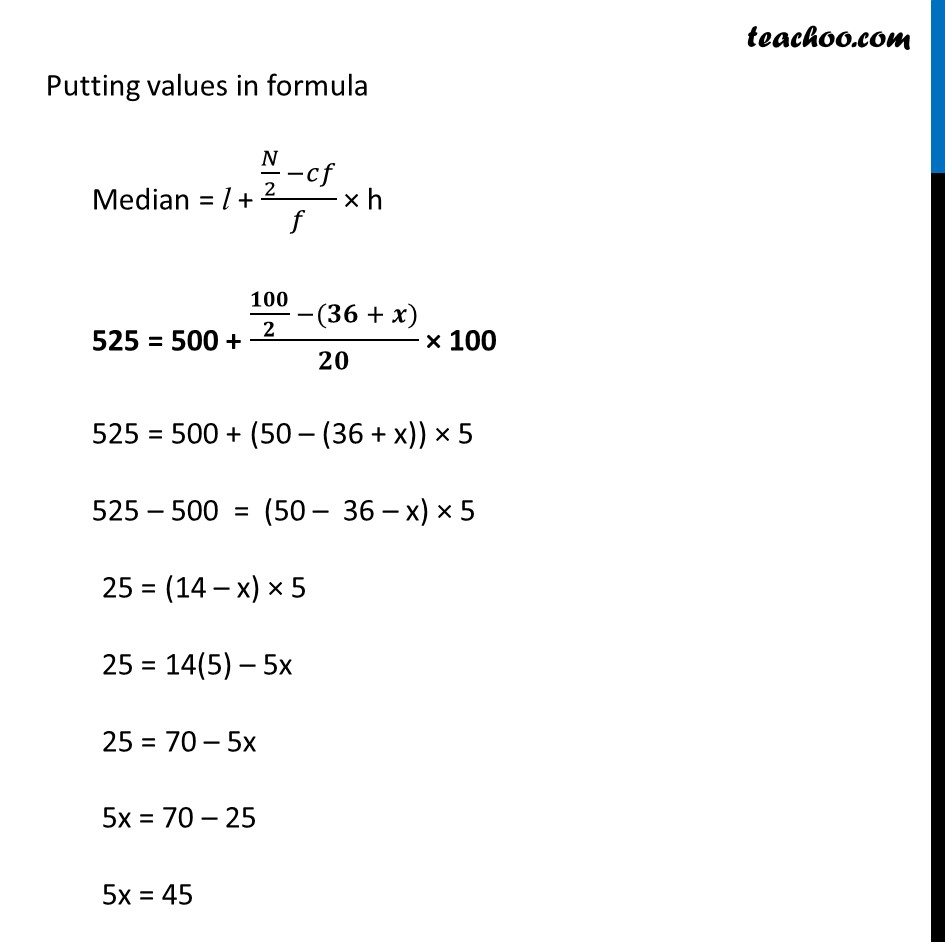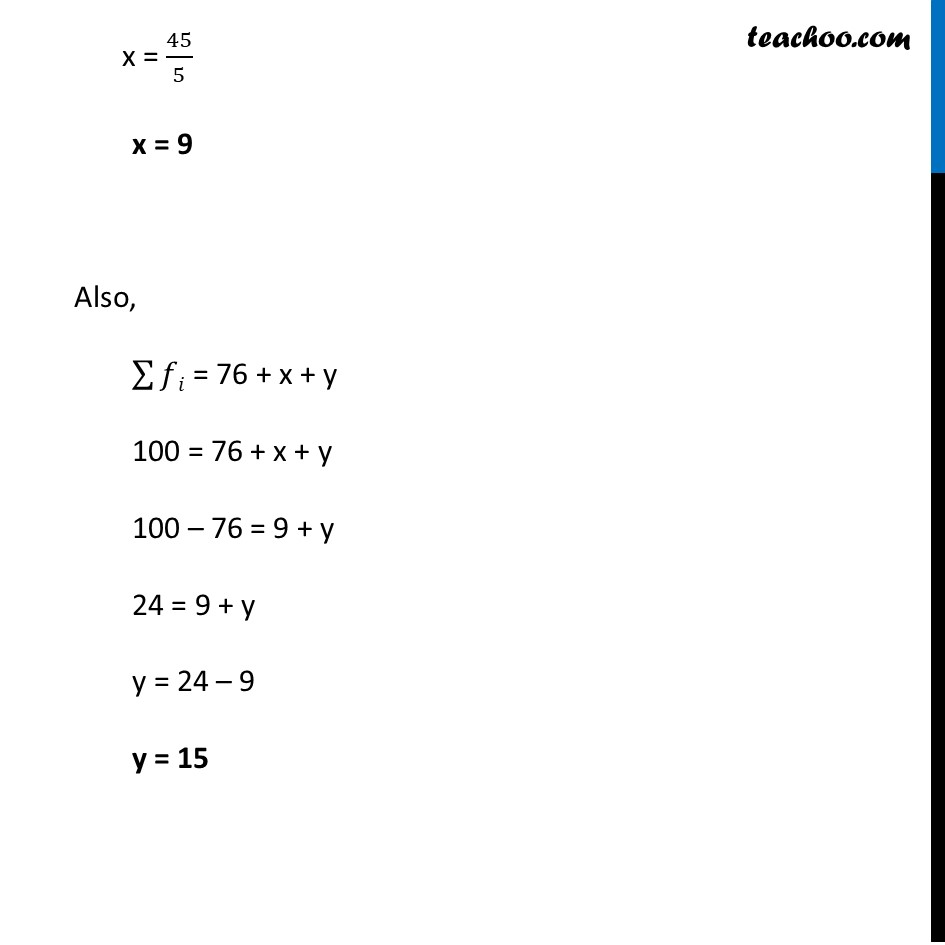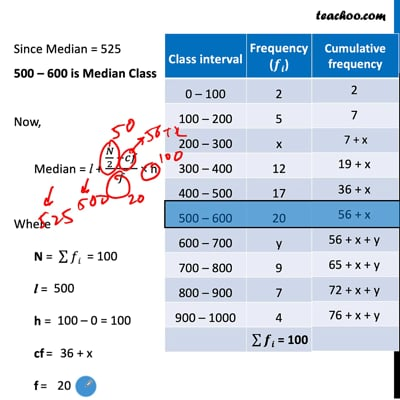This video is only available for Teachoo black users

Solve all your doubts with Teachoo Black (new monthly pack available now!)

### Transcript

Example 8 The median of the following data is 525. Find the values of x and y, if the total frequency is 100. Since Median = 525 500 – 600 is Median Class Now, Median = l + (𝑁/2 −𝑐𝑓)/𝑓 × h Where N = ∑▒𝑓𝑖 l = h = cf = f = Putting values in formula Median = l + (𝑁/2 −𝑐𝑓)/𝑓 × h 525 = 500 + (𝟏𝟎𝟎/𝟐 −(𝟑𝟔 + 𝒙))/𝟐𝟎 × 100 525 = 500 + (50 – (36 + x)) × 5 525 – 500 = (50 – 36 – x) × 5 25 = (14 – x) × 5 25 = 14(5) – 5x 25 = 70 – 5x 5x = 70 – 25 5x = 45 x = 45/5 x = 9 Also, ∑▒𝑓𝑖 = 76 + x + y 100 = 76 + x + y 100 – 76 = 9 + y 24 = 9 + y y = 24 – 9 y = 15